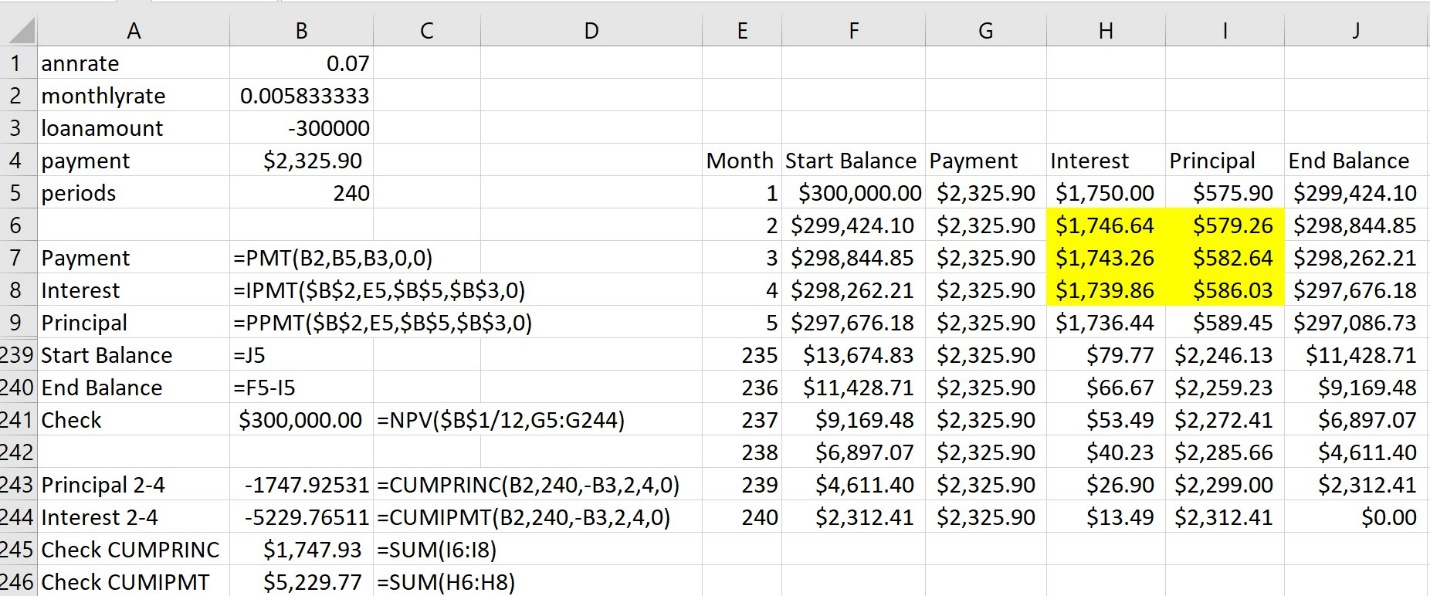# Excel Tips: Analyzing Loans in ExcelWelcome to another series of Dr. Wayne Winston’s best Excel tips! I’m Wayne Winston, Becker’s resident expert on all things Microsoft Excel. Let’s learn another helpful Excel skill that can help make your everyday accounting tasks a little bit easier.

In college, you were probably taught how to use tables in your accounting or finance textbooks to determine the monthly payment on a loan. If the monthly rate was a value not in the table, then you had to perform a messy interpolation and most likely entered the monthly payment into an Excel spreadsheet.

Wouldn’t it be better if you could simply use Excel functions to perform the computations needed to analyze loans? In this article, we will introduce you to five Excel functions that make it easy to create an amortization schedule to track our monthly payment, unpaid balance and monthly contributions to principal and interest amounts. Copy and paste this link into a new browser tab to download the Excel workbook and follow along.

To illustrate the use of these functions, we assume that you have borrowed \$300,000 for a new home. You need to make 240 monthly payments and the annual interest rate is 7%. We have hidden information for months 6-234.

##### Figure 1: Amortization schedule 20-year loan##### Excel’s Loan Functions

We now discuss the Excel functions that are useful in analyzing loans. All arguments in square brackets are optional. Let’s first look at the PMT function, which computes the periodic payment for a loan, assuming constant payments. The syntax of the PMT function is PMT(RATE,#PER,PV,[FV],[TYPE]. The square brackets indicate that the FV and TYPE arguments are optional.

• RATE is the rate per period (.07/12).
• #PER is the number of periods (240 months).
• PV is the amount of money being borrowed. Use a minus sign if you want the payment to be positive. Therefore, we use PV = -300,000.
• FV is an optional argument (assumed 0 if omitted) that is the amount of money paid (often called a balloon payment). If you are making a balloon payment, the sign of the FV should be the opposite of the sign used for PV. Thus, if we were paying \$5,000 at the end of the loan, we would enter FV= \$5,000.
• TYPE is an optional argument, with 0 or omitted used for end of period payments, and 1 used for beginning of period payments.

In cell B2, we compute the monthly rate by dividing the annual rate by 12. Then the formula =PMT(B2,B5,B3,0,0) in cell B4 computes the monthly payment (\$2,325.90.) In cell B241, we use Excel’s NPV function to show that the Net Present Value of all payments equals the loan value.

We now describe 4 other functions used to analyze loans. The arguments in these functions have the same meaning as the PMT Function.

1. IPMT(RATE,PER,#PER,PV, [FV],[TYPE]) computes the portion of the payment during PER that goes to interest. Thus, in cell H5 the formula =IPMT(\$B\$2,E5,\$B\$5,\$B\$3,0) shows that during month 1, \$1,750 of our payment went to interest.
2. PPMT(RATE,PER,#PER,PV, [FV],[TYPE]) computes the portion of the payment during PER that goes to principal. Thus, in cell I5 the formula =PPMT(\$B\$2,E5,\$B\$5,\$B\$3,0) shows that during month 1, \$575.90 of our payment went to interest. Of course, during each month columns H and I will add up to the monthly payment.
3. CUMPRINC(RATE,#PER,PV,START_PERIOD,END_PERIOD,[TYPE]) computes total contribution to principal during months between START_PERIOD and END_PERIOD inclusive. Thus, in cell B243 the formula =CUMPRINC(B2,240,-B3,2,4,0) shows that total contribution to principal during months 2-4 is \$1,747.93. In cell B245 we check this calculation by adding up actual principal contribution in months 2-4.
4. CUMIPMT(RATE,#PER,PV,START_PERIOD,END_PERIOD,[TYPE]) computes total interest payments during months between START_PERIOD and END_PERIOD inclusive. Thus, in cell B244 the formula = CUMIPMT(B2,240,-B3,2,4,0) shows that total interest payments during months 2-4 is \$5,229.77. In cell B246, we check this calculation by adding up actual principal contribution in months 2-4.

To complete the Amortization Schedule, we copy the formulas in G5:I5 to G6:I244. In column J, we compute each month’s ending balance as Start Balance-Contribution to Principal. In Column F, we compute each month’s start balance as the previous month’s ending balance.

Finally, in cell J244, our ending loan balance is \$0, and we have paid off the loan.

I hope you agree that these Excel functions make loan analysis fun! Keep visiting the Becker blog for more of my best Excel tips.

### Now Leaving Becker.com

You are leaving the Becker.com website. Once you click “continue,” you will be brought to a third-party website. Please be aware, the privacy policy may differ on the third-party website. Adtalem Global Education is not responsible for the security, contents and accuracy of any information provided on the third-party website. Note that the website may still be a third-party website even the format is similar to the Becker.com website.